# Quiz 4: Utility Maximization and Choice

a) P's utility function is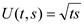His budget constraint is: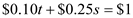Setting the Lagrange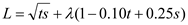Yields the first order conditions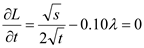...... (1)...... (2) Dividing equation (1) by equation (2), we get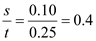Thus,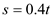...... (3) Substitution of this value of s into the budget constraintWe get,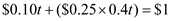Solving for t yields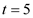Substituting the above value of t in equation (3),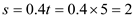As shown above, P should buy 5 twinkies and 2 cups of soda. b) P's utility when the price of twinkies was \$0.10 each is found by substituting the optimal values t = 5 and s = 2 into the utility function.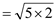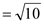Now, we will maximize P's utilityWhen the price of twinkies is \$0.40 The new budget constraint is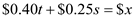Where \$ x is the increased money his mother gives him so that his utility remains at the initial level of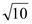. Setting the Lagrange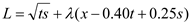Yields the first order condition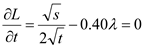...... (4)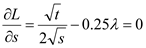...... (5) Taking the ratio of the first two terms shows that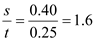Thus,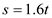...... (6) Substitution of this value of s into the budget constraint gives: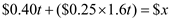Solving for t yields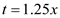Substituting the above value of t in equation (6) we get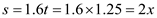The utility level when t is 1.25 x and s is 2 x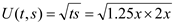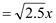Equating this utility level with his initial utility level of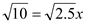We get,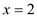As his initial allowance was \$1, his mother will have to increase his allowance by \$1.
a. Utility function is a mathematical function which gives the level of utility achieved by an individual at different amounts of commodities. Individual's utility is represented by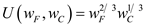The Budget constraint is given by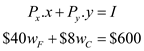Setting the Lagrange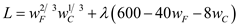The first order conditions for the Lagrange equation are given below:…… (1)…… (2) Dividing equation (1) by equation (2), the value of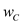can be calculated as follows:Thus,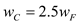Putting this value into budget constraint, the value of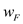can be calculated as follows:Substituting the above value of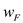in equation:To maximize her utility, she should purchase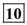bottles of French Bordeaux and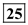bottles of California wine. b. The utility function of the individual isgiven below:When the of pricefalls to \$20, the new budget constraint is given below: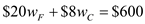The Lagrange equation is given below: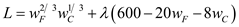The first order condition for the Lagrange equations are given below:…… (3)…… (4) Dividing equation (3) by equation (4), the value ofcan be calculated as follows:Thus,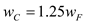Putting this value into budget constraint, the value ofcan be calculated as follows:Substituting the above value ofin equation the value of can becalculated as shown below:To maximize her utility, she should purchase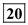bottles of French Bordeaux andbottles of California wine. c. As price of French Bordeaux decreases from \$40 to \$20 and individual can buy more of French Bordeaux she is better off in second situation.She is consuming same amount of California wine and more French Bordeaux wine. The monetary value that can be put on this utility increase is the additional money that the individual would have had to spend to purchase the additional 10 bottles of French Bordeaux at the initial price of \$40. The monetary value of utility increase is calculated below: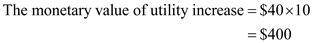Therefore, the monetary value of increase in utility is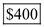.
a) The utility function of the individual is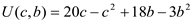As cost is not object, there does not exist a budget constraint. First order conditions of the utility function is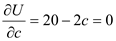...... (1)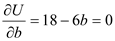...... (2) From equation (1) we get c = 10 From equation (2) we get, b = 3 The individual should consume 3 units of b and 10 units of c to maximize utility. b) The utility function of the individual is given by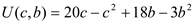As instructed by the doctor, his constraint is given by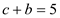Thus, b = 5 - c Substituting this in the utility function, we get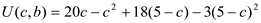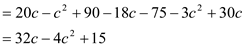The first order condition is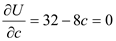Therefore,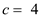Putting this back into equation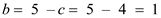The individual should consume 1 unit of b and 4 units of c to maximize his utility.